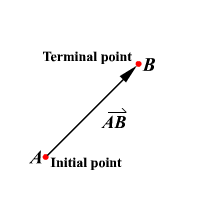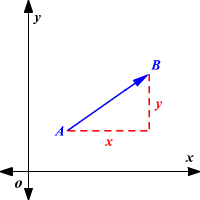# Vectors

Consider the two statements.

1. A car is moving with a speed of $100$ mph.

2. A car is moving with a speed of $100$ mph towards north.

The first statement states only the speed with which the car moves. The second statement states both the speed and direction of the car.

The second statement describes both the speed and direction of the car.

Vector

A vector is a quantity which is described by both magnitude and direction.

Quantities such as force, velocity, and acceleration that has both magnitude and direction are vectors.

The diagram shows a vector with initial point $A$ and terminal point $B$ .This vector can be represented by $\stackrel{\to }{AB}$ read as " vector $\mathrm{AB}$ . "

Component Form of a Vector

The component form of a vector combines the horizontal and vertical components.The component form of $\stackrel{\to }{AB}$ is $\left(x,y\right)$ .